# Unsteady MHD Mass Transfer Flow past a Temporarily Accelerated Semi-infinite Vertical Plate in Presence of Thermal Diffusion with Ramped Wall Temperature

Unsteady MHD Mass Transfer Flow past a Temporarily Accelerated Semi-infinite Vertical Plate in Presence of Thermal Diffusion with Ramped Wall Temperature
Department of Mathematics, Gauhati University, Guwahati-14, Assam, India

Corresponding Author Email:
kangkan22@gmail.com
Page:
241-248
|
DOI:
https://doi.org/10.18280/mmep.060212
27 August 2018
|
Accepted:
22 November 2018
|
Published:
20 June 2019
| Citation

OPEN ACCESS

Abstract:

An exact solution to the problem of transient MHD mass transfer flow past a temporarily accelerated vertical plate with ramped wall temperature has been presented. The novelty of the present work is to analyze the influence of Soret effect on the flow and transport characteristics in the presence of thermal radiation and ramped wall temperature. The governing equations are solved by Laplace transform technique in closed form. The expressions for the velocity, temperature and concentration fields as well as skin friction, rates of heat and mass transfer in terms of Nusselt number and Sherwood number are obtained in non dimensional form. Influence of the physical parameters on the above fields which involved in the problem are demonstrated through graphs. It is seen that fluid velocity and concentration increase with the increase in Soret number.

Keywords:

heat transfer, ramped temperature, thermal diffusion, thermal radiation

1. Introduction

MHD is the branch of physics that studies the behaviour of an electrically conducting fluid acted on by a magnetic field. There are numerous examples of applications of MHD principles including MHD generators, MHD pumps and MHD flow meters etc. MHD principles find its applications in Medicine and Biology also. Some applications of MHD have become a matter of interest for many researchers such as Plasma Physics, Missile Technology, and Geophysics etc. MHD may be utilized in the continuous casting process of metals to dominate instabilities and control the flow. Significant studies on MHD free convective flows have been carried out by several authors. Some of them are Alfven , Seth et al. , Das et al. , Ahmed , Ahmed and Dutta , Seth et al.  etc.

Natural flow arises in fluid when the temperature change causes density variation leading to buoyancy forces acting on the fluid. Free convection is a process of heat transfer in natural flow. The heating of rooms and buildings by use of radiator is an example of heat transfer by free convection. Radiation is the transmission of energy in the form of waves or particles through a material medium. Radiative flows are used in many industrial and environmental processes such as heating and cooling chambers, energy processes etc. It is used also for diagnosis, skin treatment, and cancer treatment etc. Due to importance of the above physical aspects, many researchers have been studied on the problems of mass transfer with radiation under different physical conditions. Some of them are Takhar et al. , Raptis and Perdikis , Alam et al. , Sattar and Kalim , Mankinde  and Mansour  etc. Investigation of problems on natural convective radiating flow of electrically conducting fluid past infinite plate becomes very interesting and fruitful when a magnetic field is applied normal to the plate. Comprehensive literature on various aspects of free convective radiative MHD flows and its applications can be found in Sattar and Maleque , Ahmed and Sarmah  and Ahmed .

The process of mass transfer due to temperature gradient is known as thermal diffusion or Soret effect. The experimental investigation of the thermal diffusion effect on mass transfer related problems was first performed by Charles Soret in 1879. Unsteady MHD free convective mass transfer flow past an infinite vertical porous plate with variable suction and Soret effect has been studied by Reddy et al. . Hossain et al.  considered the study of unsteady MHD free convection flow past a vertical plate with thermal diffusion and chemical recation. Devi and Raj  investigated thermo diffusion effects on unsteady hydromagnetic free convection flow with heat and mass transfer past a moving vertical plate with time dependent suction and heat source in a slip flow regime. Mythreye et al.  considered chemical reaction and Soret effect on MHD free convective flow past an infinite vertical porous plate with variable suction. The Soret effect on free convective unsteady MHD flow over a vertical plate with heat source investigated by Bhavana et al. .

The objective of the present work is to study the thermal diffusion effect on MHD mass transfer flow past a temporarily accelerated vertical plate with ramped wall temperature. The resulting system of the equations governing the flow is solved by adopting Laplace transform technique in closed form. Moreover, the present work is also concerned with mass transfer effects as these are encountered in separation process, removal of pollutants in many chemical reactions. However, in the present investigation, a comparison has been shown with previously published results to validate the accuracy of the present work.

2. Basic Equations

The equations governing the flow of a viscous incompressible and electrically conducting fluid in the presence of magnetic field are

Equation of continuity:

$\vec{\bigtriangledown}.\vec{q}=0$ (1)

Momentum equation:

$ρ[\frac{\partial\vec{q}}{\partial t*}+(\vec{q}.\vec{\bigtriangledown})\vec{q}]=-\vec{\bigtriangledown}p+\vec{J}\times\vec{B}+ρ\vec{g}+\mu\bigtriangledown ^2\vec{q}$  (2)

Ohm’s law:

$\vec{J}=\sigma[\vec{E}+(\vec{q}\times\vec{B})]$ (3)

Gauss’ law of magnetism:

$\vec{\bigtriangledown}.\vec{B}=0$  (4)

Energy equation:

$\rho {{C}_{p}}\left[ {{\frac{\partial N* }{\partial {{t}^{*}}}}^{*}}+\left( \vec{q}.\vec{\nabla } \right){{N}^{*}} \right]=\kappa {{\nabla }^{2}}{{N}^{*}}-\vec{\nabla }.{{\vec{q}}_{r}}$ (5)

Species continuity equation:

\frac{\partial {\vec{H}}*}{\partial t*}+(\vec{q}.\bigtriangledown)H*=D_M\bigtriangledown^2H*+\frac{D_MK_T}{T_M}\bigtriangledown^2N* (6) All the physical quantities are defined in the Nomenclature. 3. Mathematical Analysis Let us introduce a coordinate system (x*,y*,z*) with X –axis, Y –axis and Z –axis respectively along the plate, normal to the plate and width of the plate. Initially the p late and the fluid were at rest at the same temperature N'_\infty and concentration H'_\infty . At time t*>0 , the plate suddenly moves with acceleration \frac{U_0}{t_0} for 0<t*≤t_0 and for t*>t_0 the plate moves with constant speed U_0. Let \vec{q}=(u*,0,0) denotes the fluid velocity and \vec{B}=(0,B_0,0) be the applied magnetic field at the point (x*, y*, z*, t* ) in the fluid. Equation of state according to classical Boussinesq approximation is ${{\rho }_{\infty }}=\rho \left[ 1+\beta \left( {{N}^{*}}-N_{\infty }^{*} \right)+{{\beta }^{*}}\left( {{H}^{*}}-H_{\infty }^{*} \right) \right]$ (7) The governing equations of motion in terms of mass continuity, momentum, energy and species continuity are \frac{\partial u*}{\partial x*} (8) \frac{\partial u*}{\partial t*}=u\frac{\partial ^2u*}{\partial y*^2}+gβ(N*-N*_\infty) (9) \rho {{C}_{p}}\frac{\partial {{N}^{*}}}{\partial {{t}^{*}}}=\kappa \frac{{{\partial }^{2}}{{N}^{*}}}{\partial {{y}^{{{*}^{2}}}}}-\frac{\partial q_{r}^{*}}{\partial {{y}^{*}}} (10) \frac{\partial {{H}^{*}}}{\partial {{t}^{*}}}={{D}_{M}}\frac{{{\partial }^{2}}{{H}^{*}}}{\partial {{y}^{{{*}^{2}}}}}+\frac{{{D}_{M}}{{K}_{T}}}{{{T}_{M}}}\frac{{{\partial }^{2}}{{N}^{*}}}{\partial {{y}^{{{*}^{2}}}}} (11) Subject to initial and boundary conditions \begin{align} & {{u}^{*}}=0,{{N}^{*}}=N_{\infty }^{*},{{H }^{*}}=H _{\infty }^{*}\,\,\,\forall \,{{y}^{*}}>0,{{t}^{*}}\le 0 \\ & {{u}^{*}}=\frac{{{U}_{0}}}{{{t}_{0}}}{{t}^{*}},{{N}^{*}}=N_{\infty }^{*}+\left(N _{w}^{*}-N_{\infty }^{*} \right)\frac{{{t}^{*}}}{{{t}_{0}}} \\ & \,\,\,\,\,\,\,\,\,\,\,\,\,\,\,\,\,\,\,\,\,\,\,\,\,\,\,\,\,\,\,\,\,\,\,\,{{H }^{*}}=H _{w}^{*}\,\,\,\text{at}\,\,{{y}^{*}}=0,{{t}^{*}}\le {{t}_{0}} \\ & {{u}^{*}}=\frac{{{U}_{0}}}{{{t}_{0}}}{{t}^{*}},{{N}^{*}}=N_{w}^{*},{{H}^{*}}=H _{w}^{*}\,\,\,\text{at}\,\,{{y}^{*}}=0,\,{{t}^{*}}>{{t}_{0}} \\ & {{u}^{*}}\to 0,{{N}^{*}}\to N_{\infty }^{*},{{H }^{*}}\to H_{\infty }^{*}\,\,\text{as}\,\,{{y}^{*}}\to \infty ,{{t}^{*}}>0 \\ \end{align} (12) It is emphasized by Cogley et al.  that the rate of radiative flux is given by \frac{\partial q_{r}^{*}}{\partial {{y}^{*}}}=4I\left( {{N}^{*}}-N_{\infty }^{*} \right) (13) 1. I=\int\limits_{0}^{\infty }{{{\left( {{K}_{\lambda }} \right)}_{w}}}{{\left( \frac{\partial {{e}_{b\lambda }}}{\partial {{N}^{*}}} \right)}_{w}}d\lambda We have introduced some non-dimensional variables and parameters to normalize the mathematical model of the problem u=\frac{{{u}^{*}}}{{{U}_{0}}},y=\frac{{{y}^{*}}}{{{U}_{0}}{{t}_{0}}},t=\frac{{{t}^{*}}}{{{t}_{0}}},\delta =\frac{{{N}^{*}}-N_{\infty }^{*}}{N_{w}^{*}-N_{\infty }^{*}},\Phi =\frac{{{H}^{*}}-H_{\infty }^{*}}{H_{w}^{*}-H _{\infty }^{*}} \text{Gr}=\frac{\upsilon g\beta \left(N_{w}^{*}-N_{\infty }^{*} \right)}{U_{0}^{3}},\text{Gm}=\frac{\upsilon g{{\beta }^{*}}\left(H _{w}^{*}-H _{\infty }^{*} \right)}{U_{0}^{3}} \Pr =\frac{\mu {{C}_{p}}}{\kappa },R=\frac{4I\upsilon }{\rho {{C}_{p}}U_{0}^{2}},M=\frac{\sigma B_{0}^{2}\upsilon }{\rho U_{0}^{2}},Ra=\frac{U_{0}^{2}{{t}_{0}}}{\upsilon } \text{Sc}=\frac{\upsilon }{{{D}_{M}}}\text{,Sr}=\frac{{{D}_{T}}\left(N_{w}^{*}-N_{\infty }^{*} \right)}{\upsilon \left( H_{w}^{*}-H_{\infty }^{*} \right)} Non-dimensional form of the equations (9)-(11) are \frac{\partial u}{\partial t}=\frac{1}{Ra}\frac{{{\partial }^{2}}u}{\partial {{y}^{2}}}+Ra\text{Gr}\delta +Ra\text{Gm}\Phi -MRau (14) \frac{\partial \delta }{\partial t}=\frac{1}{Ra\Pr }\frac{{{\partial }^{2}}\delta }{\partial {{y}^{2}}}-RaR\delta (15) \frac{\partial \Phi }{\partial t}=\frac{1}{Ra\text{Sc}}\frac{{{\partial }^{2}}\Phi }{\partial {{y}^{2}}}+\frac{\text{Sr}}{Ra}\frac{{{\partial }^{2}}\delta }{\partial {{y}^{2}}} (16) Subject to initial and boundary conditions \begin{align} & u=0,\delta =0,\Phi =0\,\,\,\,\forall \,\,y>0,t\le 0 \\ & u=t,\delta =t,\Phi =1\,\,\text{at}\,\,y=0,0<t\le 1 \\ & u=1,\delta =1,\Phi =1\,\,\text{at}\,\,y=0,t>1 \\ & u\to 0,\delta \to 0,\Phi \to 0\,\,\text{as}\,\,y\to \infty ,t>0 \\ \end{align} (17) 4. Method of Solution On taking Laplace transforms of the equations (14) to (16) subject to conditions (17), we have obtained following differential equations \frac{{{d}^{2}}\bar{\delta }}{d{{y}^{2}}}-\left( s+RaR \right)\Pr Ra\bar{\delta }=0 (18) \frac{{{d}^{2}}\bar{\Phi }}{d{{y}^{2}}}-sRa\text{Sc}\bar{\Phi }\text{=}-\text{ScSr}\frac{{{d}^{2}}\bar{\delta }}{d{{y}^{2}}} (19) \frac{{{d}^{2}}\bar{u}}{d{{y}^{2}}}-\left( s+MRa \right)Ra\bar{u}=-R{{a}^{2}}\left( \text{Gr}\bar{\delta }+\text{Gm}\bar{\Phi } \right) (20) Subject to the boundary conditions \begin{align} & \bar{u}=\frac{1}{{{s}^{2}}}\left( 1-{{e}^{-s}} \right),\bar{\delta }=\frac{1}{{{s}^{2}}}\left( 1-{{e}^{-s}} \right),\bar{\Phi }=\frac{1}{s}\,\,\, \\ & \,\,\,\,\,\,\,\,\,\,\,\,\,\,\,\,\,\,\,\,\,\,\,\,\,\,\,\,\,\,\,\,\,\,\,\,\,\,\,\,\,\,\,\,\,\,\,\,\,\,\,\,\,\,\text{at}\,\,\,y=0 \\ & \bar{u}\to 0,\bar{\delta }\to 0,\bar{\Phi }\to 0\,\,\,\text{as}\,\,\,y\to \infty\\ \end{align} (21) The solutions of the equations (18) to (20) under the boundary conditions (21) are as follows: \bar{\delta }=\frac{1}{{{s}^{2}}}\left( 1-{{e}^{-s}} \right){{e}^{-\sqrt{s+RaR}\sqrt{\Pr Ra}y}} (22) \bar{\Phi }=\left[ \frac{1}{s}+{{L}_{1}} \right]{{e}^{-\sqrt{sRa\text{Sc}}y}}-{{L}_{1}}{{e}^{-\sqrt{s+RaR}\sqrt{\Pr Ra}y}} (23) \begin{align} & \bar{u}=\left( {{L}_{2}}+{{L}_{3}}+{{L}_{4}}+{{L}_{5}}+{{L}_{6}} \right){{e}^{-\sqrt{s+RaM}\sqrt{Ra}y}} \\ & \,\,\,\,\,-\left( {{L}_{3}}+{{L}_{6}} \right){{e}^{-\sqrt{s+RaR}\sqrt{\Pr Ra}y}}\,-\left( {{L}_{4}}+{{L}_{5}} \right){{e}^{-\sqrt{sRa\text{Sc}}y}} \\ \end{align} (24) Taking Laplace inverse transform (22)-(24), the temperature, concentration and velocity fields are derived as follows: \delta ={{\psi }_{1}}-{{\bar{\psi }}_{1}} (25) \Phi ={{\phi }_{1}}+{{\phi }_{2}}-{{\phi }_{3}} (26) u={{\omega }_{1}}+{{\omega }_{2}}+{{\omega }_{3}}+{{\omega }_{4}}+{{\omega }_{5}}-{{\omega }_{6}}-{{\omega }_{7}}-{{\omega }_{8}}-{{\omega }_{9}} (27) where {{\phi }_{1}}={{\psi }_{2}} {{\phi }_{2}}=\frac{A}{\left( \Pr -\text{Sc} \right)}\left[ \begin{align} & {{A}_{1}}\left( {{\psi }_{2}}-{{{\bar{\psi }}}_{2}} \right)+{{A}_{2}}\left( {{\psi }_{3}}-{{{\bar{\psi }}}_{3}} \right) \\ & +{{A}_{3}}\left( {{e}^{{{a}_{1}}t}}{{\psi }_{4}}-{{e}^{{{a}_{1}}\left( t-1 \right)}}{{{\bar{\psi }}}_{4}} \right) \\ \end{align} \right] {{\phi }_{3}}=\frac{A}{\left( \Pr -\text{Sc} \right)}\left[ \begin{align} & {{A}_{1}}\left( {{\psi }_{5}}-{{{\bar{\psi }}}_{5}} \right)+{{A}_{2}}\left( {{\psi }_{1}}-{{{\bar{\psi }}}_{1}} \right) \\ & +{{A}_{3}}\left( {{e}^{{{a}_{1}}t}}{{\psi }_{6}}-{{e}^{{{a}_{1}}\left( t-1 \right)}}{{{\bar{\psi }}}_{6}} \right) \\ \end{align} \right] {{\omega }_{1}}={{\psi }_{7}}-{{\bar{\psi }}_{7}} \begin{align} & {{\omega }_{2}}={{A}_{4}}\left( {{e}^{{{a}_{3}}t}}{{\psi }_{8}}-{{e}^{{{a}_{3}}\left( t-1 \right)}}{{{\bar{\psi }}}_{8}} \right)+{{A}_{5}}\left( {{\psi }_{9}}-{{{\bar{\psi }}}_{9}} \right) \\ & \,\,\,\,\,\,\,\,\,\,\,+{{A}_{6}}\left( {{\psi }_{7}}-{{{\bar{\psi }}}_{7}} \right) \\ \end{align} {{\omega }_{3}}={{A}_{7}}{{\psi }_{9}}+{{A}_{8}}{{e}^{{{a}_{5}}t}}{{\psi }_{10}} \begin{align} & {{\omega }_{4}}={{A}_{9}}\left( {{e}^{{{a}_{1}}t}}{{\psi }_{11}}-{{e}^{{{a}_{1}}\left( t-1 \right)}}{{{\bar{\psi }}}_{11}} \right)+{{A}_{10}}\left( {{e}^{{{a}_{5}}t}}{{\psi }_{10}}-{{e}^{{{a}_{5}}\left( t-1 \right)}}{{{\bar{\psi }}}_{10}} \right) \\ & +{{A}_{11}}\left( {{\psi }_{9}}-{{{\bar{\psi }}}_{9}} \right)+{{A}_{12}}\left( {{\psi }_{7}}-{{{\bar{\psi }}}_{7}} \right) \\ \end{align} \begin{align} & {{\omega }_{5}}={{A}_{13}}\left( {{e}^{{{a}_{1}}t}}{{\psi }_{11}}-{{e}^{{{a}_{1}}\left( t-1 \right)}}{{{\bar{\psi }}}_{11}} \right)+{{A}_{14}}\left( {{e}^{{{a}_{3}}t}}{{\psi }_{8}}-{{e}^{{{a}_{3}}\left( t-1 \right)}}{{{\bar{\psi }}}_{8}} \right) \\ & +{{A}_{15}}\left( {{\psi }_{9}}-{{{\bar{\psi }}}_{9}} \right)+{{A}_{16}}\left( {{\psi }_{7}}-{{{\bar{\psi }}}_{7}} \right) \\ \end{align} \begin{align} & {{\omega }_{6}}={{A}_{4}}\left( {{e}^{{{a}_{3}}t}}{{\psi }_{12}}-{{e}^{{{a}_{3}}\left( t-1 \right)}}{{{\bar{\psi }}}_{12}} \right)+{{A}_{5}}\left( {{\psi }_{5}}-{{{\bar{\psi }}}_{5}} \right) \\ & \,\,\,\,\,\,\,\,+{{A}_{6}}\left( {{\psi }_{1}}-{{{\bar{\psi }}}_{1}} \right) \\ \end{align} {{\omega }_{7}}={{A}_{7}}\left( {{\psi }_{2}}-{{{\bar{\psi }}}_{2}} \right)+{{A}_{8}}\left( {{e}^{{{a}_{5}}t}}{{\psi }_{13}}-{{e}^{{{a}_{5}}\left( t-1 \right)}}{{{\bar{\psi }}}_{13}} \right) \begin{align} & {{\omega }_{8}}={{A}_{9}}\left( {{e}^{{{a}_{1}}t}}{{\psi }_{4}}-{{e}^{{{a}_{1}}\left( t-1 \right)}}{{{\bar{\psi }}}_{4}} \right)+{{A}_{10}}\left( {{e}^{{{a}_{5}}t}}{{\psi }_{13}}-{{e}^{{{a}_{5}}\left( t-1 \right)}}{{{\bar{\psi }}}_{13}} \right) \\ & +{{A}_{11}}\left( {{\psi }_{2}}-{{{\bar{\psi }}}_{2}} \right)+{{A}_{12}}\left( {{\psi }_{3}}-{{{\bar{\psi }}}_{3}} \right) \\ \end{align} \begin{align} & {{\omega }_{9}}={{A}_{13}}\left( {{e}^{{{a}_{1}}t}}{{\psi }_{6}}-{{e}^{{{a}_{1}}\left( t-1 \right)}}{{{\bar{\psi }}}_{6}} \right)+{{A}_{14}}\left( {{e}^{{{a}_{3}}t}}{{\psi }_{12}}-{{e}^{{{a}_{3}}\left( t-1 \right)}}{{{\bar{\psi }}}_{12}} \right) \\ & +{{A}_{15}}\left( {{\psi }_{5}}-{{{\bar{\psi }}}_{5}} \right)+{{A}_{16}}\left( {{\psi }_{1}}-{{{\bar{\psi }}}_{1}} \right) \\ \end{align} 5. Skin Friction The skin friction is given by \begin{align} & \tau ={{\left[ \frac{\partial u}{\partial y} \right]}_{y=0}} \\ & \,\,\,={{\tau }_{1}}+{{\tau }_{2}}+{{\tau }_{3}}+{{\tau }_{4}}+{{\tau }_{5}}-{{\tau }_{6}}-{{\tau }_{7}}-{{\tau }_{8}}-{{\tau }_{9}} \\ \end{align} (28) where {{\tau }_{1}}={{\Omega }_{1}}-{{\bar{\Omega }}_{1}} \begin{align} & {{\tau }_{2}}={{A}_{4}}\left( {{e}^{{{a}_{3}}t}}{{\Omega }_{2}}-{{e}^{{{a}_{3}}\left( t-1 \right)}}{{{\bar{\Omega }}}_{2}} \right)+{{A}_{5}}\left( {{\Omega }_{3}}-{{{\bar{\Omega }}}_{3}} \right) \\ & \,\,\,\,\,\,\,+{{A}_{6}}\left( {{\Omega }_{1}}-{{{\bar{\Omega }}}_{1}} \right) \\ \end{align} {{\tau }_{3}}={{A}_{7}}{{\Omega }_{3}}+{{A}_{8}}{{e}^{{{a}_{5}}t}}{{\Omega }_{4}} \begin{align} & {{\tau }_{4}}={{A}_{9}}\left( {{e}^{{{a}_{1}}t}}{{\Omega }_{5}}-{{e}^{{{a}_{1}}\left( t-1 \right)}}{{{\bar{\Omega }}}_{5}} \right)+{{A}_{10}}\left( {{e}^{{{a}_{5}}t}}{{\Omega }_{4}}-{{e}^{{{a}_{5}}\left( t-1 \right)}}{{{\bar{\Omega }}}_{4}} \right) \\ & +{{A}_{11}}\left( {{\Omega }_{3}}-{{{\bar{\Omega }}}_{3}} \right)+{{A}_{12}}\left( {{\Omega }_{1}}-{{{\bar{\Omega }}}_{1}} \right) \\ \end{align} \begin{align} & {{\tau }_{5}}={{A}_{13}}\left( {{e}^{{{a}_{1}}t}}{{\Omega }_{5}}-{{e}^{{{a}_{1}}\left( t-1 \right)}}{{{\bar{\Omega }}}_{5}} \right)+{{A}_{14}}\left( {{e}^{{{a}_{3}}t}}{{\Omega }_{2}}-{{e}^{{{a}_{3}}\left( t-1 \right)}}{{{\bar{\Omega }}}_{2}} \right) \\ & +{{A}_{15}}\left( {{\Omega }_{3}}-{{{\bar{\Omega }}}_{3}} \right)+{{A}_{16}}\left( {{\Omega }_{1}}-{{{\bar{\Omega }}}_{1}} \right) \\ \end{align} \begin{align} & {{\tau }_{6}}={{A}_{4}}\left( {{e}^{{{a}_{3}}t}}{{\Omega }_{6}}-{{e}^{{{a}_{3}}\left( t-1 \right)}}{{{\bar{\Omega }}}_{6}} \right)+{{A}_{5}}\left( {{\Omega }_{7}}-{{{\bar{\Omega }}}_{7}} \right) \\ & \,\,\,\,\,\,\,\,\,+{{A}_{6}}\left( {{\Omega }_{8}}-{{{\bar{\Omega }}}_{8}} \right) \\ \end{align} {{\tau }_{7}}={{A}_{7}}\left( {{\Omega }_{9}}-{{{\bar{\Omega }}}_{9}} \right)+{{A}_{8}}\left( {{e}^{{{a}_{5}}t}}{{\Omega }_{10}}-{{e}^{{{a}_{5}}\left( t-1 \right)}}{{{\bar{\Omega }}}_{10}} \right) \begin{align} & {{\tau }_{8}}={{A}_{9}}\left( {{e}^{{{a}_{1}}t}}{{\Omega }_{11}}-{{e}^{{{a}_{1}}\left( t-1 \right)}}{{\Omega }_{11}} \right)+{{A}_{10}}\left( {{e}^{{{a}_{5}}t}}{{\Omega }_{10}}-{{e}^{{{a}_{5}}\left( t-1 \right)}}{{{\bar{\Omega }}}_{10}} \right) \\ & +{{A}_{11}}\left( {{\Omega }_{9}}-{{{\bar{\Omega }}}_{9}} \right)+{{A}_{12}}\left( {{\Omega }_{12}}-{{{\bar{\Omega }}}_{12}} \right) \\ \end{align} \begin{align} & {{\tau }_{9}}={{A}_{13}}\left( {{e}^{{{a}_{1}}t}}{{\Omega }_{13}}-{{e}^{{{a}_{1}}\left( t-1 \right)}}{{{\bar{\Omega }}}_{13}} \right)+{{A}_{14}}\left( {{e}^{{{a}_{3}}t}}{{\Omega }_{6}}-{{e}^{{{a}_{3}}\left( t-1 \right)}}{{{\bar{\Omega }}}_{6}} \right) \\ & +{{A}_{15}}\left( {{\Omega }_{7}}-{{{\bar{\Omega }}}_{7}} \right)+{{A}_{16}}\left( {{\Omega }_{8}}-{{{\bar{\Omega }}}_{8}} \right) \\ \end{align} 6. Nusselt Number The heat transfer rate is given by \begin{align} & \text{Nu}=-{{\left[ \frac{\partial \delta }{\partial y} \right]}_{y=0}} \\ & \,\,\,\,\,\,\,=-\left( {{\Omega }_{8}}-{{{\bar{\Omega }}}_{8}} \right) \\ \end{align} (29) 7. Sherwood Number The mass transfer rate is expressed as \begin{align} & \text{Sh}=-{{\left[ \frac{\partial \Phi }{\partial y} \right]}_{y=0}} \\ & \,\,\,\,\,\,\,=-\left( {{{\bar{\phi }}}_{1}}+{{{\bar{\phi }}}_{2}}-{{{\bar{\phi }}}_{3}} \right) \\ \end{align} (30) where {{\bar{\phi }}_{1}}={{\Omega }_{9}} {{\bar{\phi }}_{2}}=\frac{A}{\left( \Pr -\text{Sc} \right)}\left[ \begin{align} & {{A}_{1}}\left( {{\Omega }_{9}}-{{{\bar{\Omega }}}_{9}} \right)+{{A}_{2}}\left( {{\Omega }_{12}}-{{{\bar{\Omega }}}_{12}} \right) \\ & +{{A}_{3}}\left( {{e}^{{{a}_{1}}t}}{{\Omega }_{11}}-{{e}^{{{a}_{1}}\left( t-1 \right)}}{{{\bar{\Omega }}}_{11}} \right) \\ \end{align} \right] {{\bar{\phi }}_{3}}=\frac{A}{\left( \Pr -\text{Sc} \right)}\left[ \begin{align} & {{A}_{1}}\left( {{\Omega }_{7}}-{{{\bar{\Omega }}}_{7}} \right)+{{A}_{2}}\left( {{\Omega }_{8}}-{{{\bar{\Omega }}}_{8}} \right) \\ & +{{A}_{3}}\left( {{e}^{{{a}_{1}}t}}{{\Omega }_{13}}-{{e}^{{{a}_{1}}\left( t-1 \right)}}{{{\bar{\Omega }}}_{13}} \right) \\ \end{align} \right] 8. Results and Discussion Numerical calculations have been carried out for the velocity, temperature and concentration fields, viscous drag, Nusselt number and Sherwood number at the plate by assigning some arbitrarily chosen specific values to the physical parameters like magnetic parameter, radiation parameter, Soret number, ramped parameter, normal coordinate and time. Throughout our investigation, the values of the Prandtl number and Schmidt number have been kept fixed at 0.71 and 0.60 which corresponds to air and water vapour (H2O) respectively with air as the diffusing medium. Figures 1 – 4 exhibit the variation of velocity profiles under the influence of M and Sr versus normal coordinate y for the cases 0<t≤1 and t>0. All the figures indicate that the effect of buoyancy force is more pronounced near the plate and its effect gets nullified in the fluid region far away from the plate. Figures 1 and 2 depict that the fluid velocity decreases comprehensively for increasing magnetic parameter. In other words the fluid motion is decelerated due to imposition of the transverse magnetic field. This observation is consistent with the physical fact that a magnetic body force (called Lorentz force) develops due to the interaction of fluid velocity and magnetic field which serves as a resistive force to the flow and as a consequence fluid flow gets decelerated. The Soret number Sr represents the thermal diffusion effect in the present work. It is observed from the figures 3 and 4 that the velocity of the fluid gets accelerated due to increasing Soret number. It indicates that the thickness of the velocity boundary layer gets increased due to thermal diffusion and hence thereby increases the fluid motion. The effect of the radiation parameter R on the temperature profile is shown in Figure 6. This figure shows that the temperature decreases as the radiation parameter R increases. This result qualitatively agrees with expectations, since the effect of radiation is to decrease the rate of energy transport to the fluid, thereby reducing the fluid temperature. It is noticed from Figure 5 that the temperature drops due to ramped parameter for both ramped and isothermal wall temperature. The influence of Soret and Schmidt numbers on the concentration profiles is depicted in Figures 7 and 8 respectively. It is observed from Figure 7 that an increase in the Soret number results in an increase in the concentration level of the fluid. Figure 8 show that there is a substantial fall in the concentration level when Schmidt number Sc is increased for both ramped as well as isothermal wall temperature. This observation is in consistent to the physical fact that an increase in Schmidt number means a decrease in mass diffusivity which results in a fall in the concentration level of the fluid. The effects of the radiation parameter R and the ramped parameter Ra on the Nusselt number have been presented in Figures 9 and 10. These figures show that Nu increases steadily from its minimum value Nu = 0 at t = 0 as time progresses. Physically, the heat transfer rate is stabilized for t > 1. The change of behaviour of the Sherwood number versus t under the influence of Soret number and ramped parameter is illustrated in Figures 11 and 12. These figures show that the rate of mass transfer from the plate to the fluid is increased under the effects of Soret number and ramped parameter. ## figure_1.png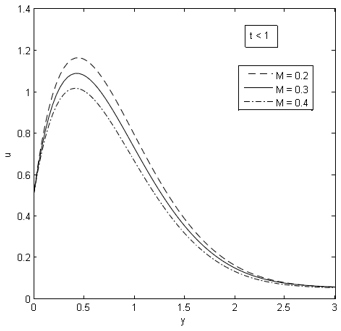Figure 1. Magnetic parameter on velocity profile for R=1, Ra=1, Sc=0.6, Sr=1, Pr=0.71, Gr=10, Gm=5, t=0.5 ## figure_2.png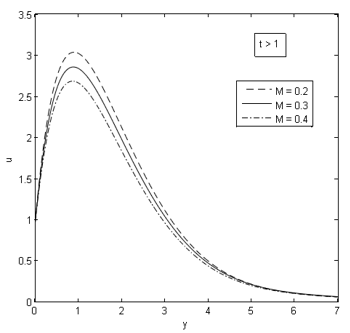Figure 2. Magnetic parameter on velocity profile fo R=1, Ra=1, Sc=0.6, Sr=1, Pr=0.71, Gr=10, Gm=5, t=2 ## figure_3.pngFigure 3. Soret number on velocity profile for R=1, Ra=1, Sc=0.6, M=1, Pr=0.71, Gr=10, Gm=5, t=0.5 ## figure_4.png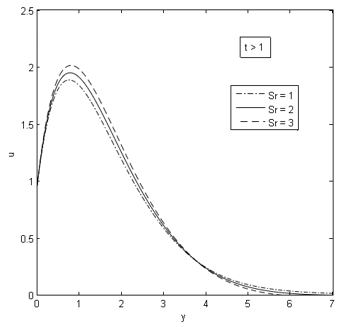Figure 4. Soret number on velocity profile for R=1, Ra=1, Sc=0.6, M=1, Pr=0.71, Gr=10, Gm=5, t=2 ## figure_5.png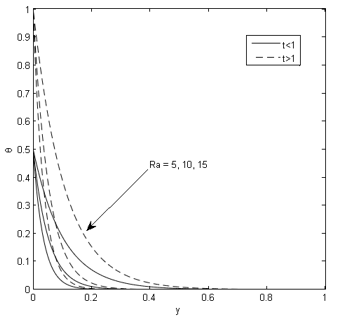Figure 5. Temperature \delta versus y for R=1, Pr=0.71, t=0.5, t=2 ## figure_6.pngFigure 6. Temperature \delta versus y for Ra=1, Pr=0.71, t=0.5, t=2 ## figure_7.png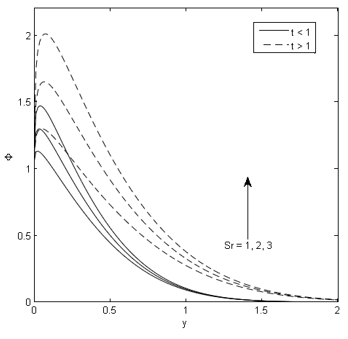Figure 7. Concentration \varPhi versus y for Ra=1, R=1, Sc=0.6, Pr=0.71, t=0.5 ## figure_8.pngFigure 8. Concentration \varPhi versus y for Ra=1, R=1, Sc=0.6, Pr=0.71, t=2 ## figure_9.png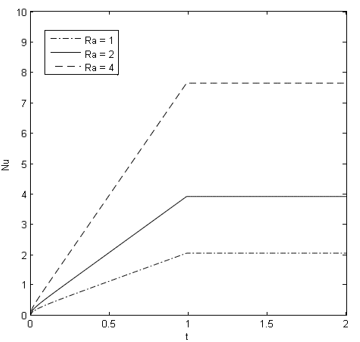Figure 9. Nusselt number Nu versus t for R=1,\text{Pr}=0.71 ## figure_10.png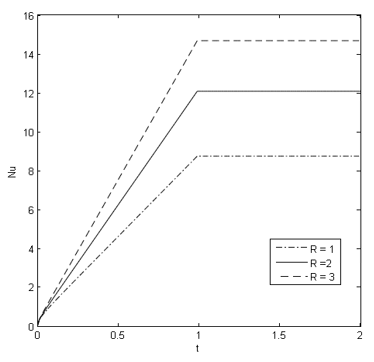Figure 10. Nusselt number Nu versus t for Ra=1,\text{Pr}=0.71 ## figure_11.pngFigure 11. Sherwood number Sh versus t for Ra=1,R=1,\text{Sc}=0.6,\text{Pr}=0.71 ## figure_12.png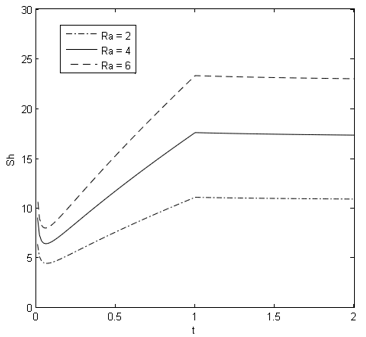Figure 12. Sherwood number Sh versus t for \text{Sr}=1,R=1,\text{Sc}=0.6,\text{Pr}=0.71 9. Comparison ## figure_13.png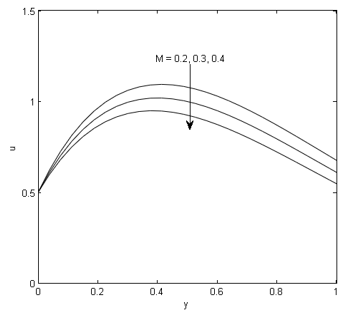Figure 13. Velocity profile for the variation in M In order to check the accuracy of our results, one of our results is compared with the work of Ahmed  which we have considered. Their work concerns with heat transfer flow with magnetic field effect whereas our work is concerned with mass transfer hydromagnetic flow in presence of thermal diffusion effect. To make a comparison with the work of Ahmed , velocity profile in the absence of mass transfer effect (i.e. Gm=0) is drawn and represented in Figure 13. Figure 13 of our work has been compared with Figure 1 of Ahmed  and it is found that both the figures uniquely indicate that fluid velocity decreases due to increasing magnetic parameter. Hence there is a good agreement between the results obtained by Ahmed  and that by the present authors. 10. Conclusions The outcomes of the present investigation are: • Fluid motion is decelerated due to the application of magnetic field. • Fluid velocity accelerates due to thermal diffusion effect. • Fluid temperature drops with the increase in ramped parameter. • The rate of mass transfer at the plate is increased due to the effect of thermal diffusion. • The rate of heat transfer is increased due to radiation. Nomenclature \vec{B} Magnetic induction vector {{B}_{0}} Strength of the applied magnetic field {{C}_{p}} Specific heat at constant pressure H* Species concentration H _{\infty }{*} Species concentration at free stream H _{w}{*} Species concentration at the plate {{D}_{M}} Mass diffusion coefficient \vec{E} Electric field intensity vector erfc Complementary error function erf Error function \vec{g} Gravitational acceleration vector g Acceleration due to gravity \text{Gr} Thermal Grashof number \text{Gm} Solutal Grashof number \vec{J} Current density vector {{K}_{T}} Thermal diffusion ratio {{K}_{\lambda }} Absorption coefficient M Magnetic parameter \text{Nu} Nusselt number p Fluid pressure \Pr Prandtl number \vec{q} Fluid velocity vector {{\vec{q}}_{r}} Radiative heat flux vector R Radiation parameter Ra Ramped parameter s Laplace transforms parameter \text{Sc} Schmidt number \text{Sr} Soret number \text{Sh} Sherwood number {{T}_{M}} Mean fluid temperature {{H}{*}} Fluid temperature H _{w}{*} Fluid temperature at the plate H_{\infty }{*} Fluid temperature at free stream {{t}^{*}} Dimensional time t Dimensionless time t0 Characteristic time {{u}{*}} X component of \vec{q} u Non dimensional fluid velocity {{U}_{0}} Plate velocity y Non dimensional y coordinate Greek symbols {{U}_{0}} Fluid density \mu Coefficient of viscosity \kappa Thermal conductivity \sigma Electrical conductivity \upsilon Kinematic viscosity \delta Dimensionless temperature \Phi Dimensionless concentration \Phi Dimensionless skin friction \beta Coefficient of volume expansion for heat transfer {{\beta }^{*}} Coefficient of volume expansion for mass transfer APPENDIX ${{L}_{1}}=\frac{A\left( s+RaR \right)\left( 1-{{e}^{-s}} \right)}{{{s}^{2}}\left( s\Pr -s\text{Sc + B} \right)},{{L}_{2}}=\frac{1}{{{s}^{2}}}\left( 1-{{e}^{-s}} \right)$ ${{L}_{3}}=\frac{Ra\text{Gr}\left( 1-{{e}^{-s}} \right)}{{{s}^{2}}\left( s\Pr -s-MRa\text{ + B} \right)},{{L}_{4}}=\frac{Ra\text{Gm}}{s\left( s\text{Sc}-s-MRa \right)}$ ${{L}_{5}}=\frac{ARa\text{Gm}\left( s+RaR \right)\left( 1-{{e}^{-s}} \right)}{{{s}^{2}}\left( s\Pr -s\text{Sc}+B \right)\left( s\text{Sc}-s-MRa \right)}$ ${{L}_{6}}=\frac{ARa\text{Gm}\left( s+RaR \right)\left( 1-{{e}^{-s}} \right)}{{{s}^{2}}\left( s\Pr -s\text{Sc}+B \right)\left( s\Pr -s-MRa+B \right)}$ $A=\text{ScSrPr},B=RaR\Pr$ \begin{align} & {{a}_{1}}=-\frac{B}{\Pr -\text{Sc}},{{a}_{2}}=\frac{Ra\text{Gr}}{\Pr -1},{{a}_{3}}=\frac{MRa-B}{\Pr -1} \\ & {{a}_{4}}=\frac{Ra\text{Gm}}{\text{Sc}-1},{{a}_{5}}=\frac{RaM}{\text{Sc}-1},{{a}_{6}}=\frac{A}{\Pr -\text{Sc}},{{a}_{7}}=\frac{Ra\text{Gm}}{\Pr -1} \\ \end{align} \begin{align} & {{A}_{1}}=-\frac{{{a}_{1}}+RaR}{a_{1}^{2}},{{A}_{2}}=-\frac{RaR}{{{a}_{1}}},{{A}_{3}}=-{{A}_{1}} \\ & {{A}_{4}}=\frac{{{a}_{2}}}{a_{3}^{2}},{{A}_{5}}=-{{A}_{4}},{{A}_{6}}=-\frac{{{a}_{2}}}{{{a}_{3}}},{{A}_{7}}=-\frac{{{a}_{4}}}{{{a}_{5}}} \\ \end{align} ${{A}_{8}}=\frac{{{a}_{4}}}{{{a}_{5}}},{{A}_{9}}=\frac{{{a}_{4}}{{a}_{6}}\left( {{a}_{1}}+RaR \right)}{a_{1}^{2}\left( {{a}_{1}}-{{a}_{5}} \right)},{{A}_{10}}=\frac{{{a}_{4}}{{a}_{6}}\left( {{a}_{5}}+RaR \right)}{a_{5}^{2}\left( {{a}_{5}}-{{a}_{1}} \right)}$ \begin{align} & {{A}_{11}}=-\left( {{A}_{9}}+{{A}_{10}} \right),{{A}_{12}}=\frac{{{a}_{4}}{{a}_{6}}RaR}{{{a}_{1}}{{a}_{5}}},{{A}_{13}}=\frac{{{a}_{6}}{{a}_{7}}\left( {{a}_{1}}+RaR \right)}{a_{1}^{2}\left( {{a}_{1}}-{{a}_{3}} \right)} \\ & {{A}_{14}}=\frac{{{a}_{6}}{{a}_{7}}\left( {{a}_{3}}+RaR \right)}{a_{3}^{2}\left( {{a}_{3}}-{{a}_{1}} \right)},{{A}_{15}}=-\left( {{A}_{13}}+{{A}_{14}} \right),{{A}_{16}}=\frac{{{a}_{6}}{{a}_{7}}RaR}{{{a}_{1}}{{a}_{3}}} \\ \end{align} \begin{aligned} \psi_{1}=f(\operatorname{Pr} R& a, \operatorname{Ra} R, y, t ), \overline{\psi}_{1} \\ &=f(\operatorname{Pr} R a, \operatorname{Ra} R, y, t-1) H(t-1) \end{aligned} \psi_{2}=\operatorname{erfc}\left(\frac{y \sqrt{R a \mathrm{Sc}}}{2 \sqrt{t}}\right), \overline{\psi}_{2}=\operatorname{erfc}\left(\frac{y \sqrt{R a \mathrm{Sc}}}{2 \sqrt{t-1}}\right) H(t-1) \psi_{3}=\lambda(\operatorname{Sc} R a, y, t), \overline{\psi}_{3}=\lambda(\operatorname{Sc} R a, y, t-1) H(t-1) \psi_{4}=\psi\left(R a \mathrm{Sc}, a_{1}, y, t\right), \overline{\psi}_{4}=\psi\left(\operatorname{RaSc}, a_{1}, y, t-1\right) H(t-1) \begin{aligned} \psi_{5}=\psi(\operatorname{Pr} R& a, R a R, y, t ), \psi_{5} \\ &=\psi(\operatorname{Pr} R a, \operatorname{Ra} R, y, t-1) H(t-1) \end{aligned} \begin{aligned} \psi_{6}=\psi\left(\operatorname{Pr} R a, a_{1}+\right.& R a R, y, t ), \overline{\psi}_{6} \\=& \psi\left(\operatorname{Pr} R a, a_{1}+R a R, y, t-1\right) H(t-1) \end{aligned} \psi_{7}=f(R a, M R a, y, t), \overline{\psi}_{7}=f(R a, M R a, y, t-1) H(t-1) \begin{aligned} \psi_{8}=\psi\left(R a, a_{3}+\right.& M R a, y, t ), \psi_{8} \\=& \psi\left(R a, a_{3}+M R a, y, t-1\right) H(t-1) \end{aligned} \psi_{9}=\psi(R a, M R a, y, t), \overline{\psi}_{9}=\psi(R a, M R a, y, t-1) H(t-1) \begin{aligned} \psi_{10}=\psi\left(R a, a_{5}\right.&+M R a, y, t ), \overline{\psi}_{10} \\=& \psi\left(R a, a_{5}+M R a, y, t-1\right) H(t-1) \end{aligned} \begin{aligned} \psi_{11}=\psi\left(R a, a_{1}+M R a,\right.& y, t ), \overline{\psi}_{11} \\=& \psi\left(R a, a_{1}+M R a, y, t-1\right) H(t-1) \end{aligned} \begin{aligned} \psi_{12}=\psi\left(\operatorname{Pr} R a, a_{3}+\right.& R a R, y, t ), \overline{\psi}_{12} \\=& \psi\left(\operatorname{Pr} R a, a_{3}+R a R, y, t-1\right) H(t-1) \end{aligned} \begin{aligned} \psi_{13}=& \psi\left(\text {RaSc}, a_{5}, y, t\right), \psi_{13} \\ &=\psi\left(\operatorname{Rasc}, a_{5}, y, t-1\right) H(t-1) \end{aligned} \Omega_{1}=F(R a, R a M, t), \overline{\Omega}_{1}=F(R a, R a M, t-1) H(t-1) \begin{aligned} \Omega_{2}=& \Omega\left(R a, a_{3}+M R a, t\right), \overline{\Omega}_{2} \\ &=\Omega\left(R a, a_{3}+M R a, t-1\right) H(t-1) \end{aligned} \Omega_{3}=\Omega(R a, R a M, t), \overline{\Omega}_{3}=\Omega(R a, R a M, t-1) H(t-1) \begin{aligned} \Omega_{4}=& \Omega\left(R a, a_{5}+M R a, t\right), \overline{\Omega}_{4} \\ &=\Omega\left(R a, a_{5}+M R a, t-1\right) H(t-1) \end{aligned} \begin{aligned} \Omega_{5}=& \Omega\left(R a, a_{1}+M R a, t\right), \overline{\Omega}_{5} \\ &=\Omega\left(R a, a_{1}+M R a, t-1\right) H(t-1) \end{aligned} \begin{aligned} \Omega_{6}=\Omega\left(\operatorname{Pr} R a, a_{3}+\right.& R a R, t ), \overline{\Omega}_{6} \\=& \Omega\left(\operatorname{Pr} R a, a_{3}+R a R, t-1\right) H(t-1) \end{aligned} \Omega_{7}=\Omega(\operatorname{Pr} R a, \operatorname{RaR}, t), \overline{\Omega}_{7}=\Omega(\operatorname{Pr} R a, \operatorname{RaR}, t-1) H(t-1)

$\Omega_{8}=F\left(\operatorname{Pr} R a, \operatorname{RaR}, \overline{\Omega}_{8}\right.$$=F(\operatorname{Pr} R a, \operatorname{Ra} R, t-1) H(t-1)$

\begin{aligned} \Omega_{9}=-\sqrt{\frac{R a S_{\mathrm{C}}}{\pi t}}, & \Omega_{10}=\Omega\left(\operatorname{RaSc}, a_{5}, t\right), \overline{\Omega}_{10} \\ &=\Omega\left(\operatorname{RaSc}, a_{5}, t-1\right) H(t-1) \end{aligned}

$\Omega_{11}=\Omega\left(\text {RaSc}, a_{1}, t\right), \overline{\Omega}_{11}=\Omega\left(\text {RaSc}, a_{1}, t-1\right) H(t-1)$

$\Omega_{12}=L(\operatorname{RaSc}, t), \overline{\Omega}_{12}=L(\operatorname{RaSc}, t-1) H(t-1)$

\begin{aligned} \Omega_{13}=\Omega\left(\operatorname{Pr} R a, a_{1}+R a R, t\right), \overline{\Omega}_{13} & \\=& \Omega\left(\operatorname{Pr} R a, a_{1}+R a R, t-1\right) H(t-1) \end{aligned}

$\psi(\xi, \eta, y, t)=\frac{1}{2}\left[\begin{array}{c}{e^{\sqrt{\xi \eta} \eta} \operatorname{erf} c\left(\frac{y}{2} \sqrt{\frac{\xi}{t}}+\sqrt{\eta t}\right)} \\ {+e^{-\sqrt{\xi \eta} y} \operatorname{erfc}\left(\frac{y}{2} \sqrt{\frac{\xi}{t}}-\sqrt{\eta t}\right)}\end{array}\right]$

$f(\xi, \eta, y, t)=\left(\frac{t}{2}+\frac{y}{4} \sqrt{\frac{\xi}{\eta}}\right) e^{\sqrt{\xi \eta} y} \operatorname{erfc}+\left(\frac{t}{2}-\frac{y}{4} \sqrt{\frac{\xi}{\eta}}\right) e^{-\sqrt{\xi \eta} y} \operatorname{erfc}\left(\frac{y}{2} \sqrt{\frac{\xi}{t}}-\sqrt{\eta t}\right)$

$\lambda(\xi, y, t)=\left(t+\frac{y^{2} \xi}{2}\right) \operatorname{erf} c\left(\frac{y}{2} \sqrt{\frac{\xi}{t}}\right)-\frac{y \sqrt{\xi t}}{\sqrt{\pi}} e^{-\frac{\xi y^{2}}{4 t}}$

$\Omega(\xi, \eta, t)=-\left[\sqrt{\frac{\xi}{\pi t}} e^{-\eta t}+\sqrt{\xi \eta} e r f(\sqrt{\eta t})\right]$

$F(\xi, \eta, t)=-\left[\begin{array}{c}{\frac{1}{2} \sqrt{\frac{\xi}{\eta}} \operatorname{erf}(\sqrt{\eta t})+} \\ {t \sqrt{\xi \eta} \operatorname{erf}(\sqrt{\eta t})+\sqrt{\frac{\xi t}{\pi}} e^{-\eta t} ]}\end{array}\right.$

$L(\xi, t)=-2 \sqrt{\frac{\xi t}{\pi}}$

$H(t-1)=\left\{\begin{array}{ll}{0,} & {t<1} \\ {1,} & {t>1}\end{array} \text { is the unit step function }\right.$

References

 Alfven H. (1942). Existence of electromagnetic hydrodynamic waves. Nature 150: 405–406. http://dx.doi.org/10.1038/150405d0

 Seth GS, Ansari MS, Nandkeolyan R. (2011). MHD natural convection flow with radiative heat transfer past an impulsively moving plate with ramped wall temperature. Heat and Mass Transfer 47(5): 551–561. http://dx.doi.org/10.1007/s00231-010-0740-1

 Das S, Jana M, Jana NR. (2011). Radiation effect on natural convection near a vertical plate embedded in porous medium with ramped wall temperature. Open J. Fluid Dynamics 1: 1–11. http://dx.doi.org/10.4236/ojfd.2011

 Ahmed N. (2015). MHD transient flow past a suddenly accelerated infinite vertical plate with ramped wall temperature in presence of thermal radiation. Bull. of Allahabad Math. Soc. 30(1): 95–118.

 Ahmed N, Dutta M. (2014). Analytical analysis of MHD transient flow past a suddenly started infinite vertical plate with ramped wall temperature and thermal radiation. J. Heat Transfer 136(4): 1–8. http://dx.doi.org/10.1115/1.4026052

 Seth GS, Kumbhakar B, Sharma R. (2016). Unsteady MHD free convection flow with Hall effect of a radiating and heat absorbing fluid past a moving vertical plate with variable ramped temperature. J. of Egyptian Math. Soc. 24: 471–478. http://dx.doi.org/10.1016/j.joems.2015.07.007

 Takhar HS, Gorla RSR, Soundalgekar VM. (1996). Radiation effects on MHD free convection flow of a radiating gas past a semi-infinite vertical plate. Int. J. Numer. Meth. for Heat and Fluid Flow 6(2): 77–83.

 Raptis A, Perdikis C. (1999). Radiation and free convection flow past a moving plate. Appl. Mech. and Engg. 4(4): 817–821.

 Alam MS, Rahman MM, Sattar MA. (2006). Free convection heat and mass transfer flow past an inclined surface with heat generation. Int. J. Sci. Tech. 11(4): 1-8.

 Sattar MA, Kalim H. (1996). Unsteady free-convection interaction with thermal radiation in a boundary layer flow past a vertical porous plate. J. Math. Phys. 30: 25-37.

 Makinde OD. (2005). Free convection flow with thermal radiation and mass transfer past a moving vertical porous plate. Int. Comm. Heat Mass Transf. 32(10): 1411-1419. http://dx.doi.org/10.1016/j.icheatmasstransfer.2005.07.005

 Mansour MA. (1990). Radiative and free convection effects on the oscillating flow past a vertical plate. AstroPhysics and Science 166(2): 269-275.

 Sattar MA, Meleque MA. (2000). Unsteady MHD natural convection flow along an accelerated porous plate with Hall current and mass transfer in a rotating porous medium. J. of Energy Heat Mass Transfer 22: 67-72.

 Ahmed N, Sarmah HK. (2009). Thermal radiation effect on a transient MHD flow with mass transfer past an impulsively fixed infinite vertical plate. Int. J. Appl. Math. And Mech. 5(5): 87-98.

 Ahmed N. (2012). Soret and radiation effects on transient MHD free convection from an impulsively started infinite vertical plate. J. Heat Transfer 134(6): 1–9. http://dx.doi.org/10.1115/1.4005749

 Ramana Reddy GV, Ramana Murthy Ch.V, Bhaskar Reddy N. (2011). Unsteady MHD free convective mass transfer flow past an infinite vertical porous plate with variable suction and Soret effect. Int. J. of Appl. Math and Mech 7(21): 70-84.

 Hossain MS, Samanth N, Ahmed SF. (2015). Study of Unsteady MHD free convection flow past a vertical plate with thermal diffusion and chemical reaction. Int. J. of P. Math. Sc. 5(1): 206-215.

 Devi SP, Anjali Raj JWS. (2011). Thermo diffusion effects on unsteady hydro magnetic free convection flow with heat and mass transfer past a moving vertical plate with time dependent suction and heat source in a slip flow regime. Int. J. of Appl. Math and Mech. 7: 20-51.

 Mythreye A, Balamurugan KS. (2017). Chemical reaction and Soret effect on MHD free convective flow past an infinite vertical porous plate with variable suction. Int. J. of Chem. Engg. Res. 9: 51-62.

 Bhavana M, Chenna Kesavaiah D, Sudhakaraiah A. (2013). The Soret effect on free convective unsteady MHD flow over a vertical plate with heat source. Int. J. of Innovative Research Sci. Eng. and Tech. 5: 1617-1628.

 Cogley LCA, Vincenti GW, Gilles SE. (1968). Differential approximation for radiative heat transfer in a Gray gas near equilibrium. Amer. Inst. Aero. Astro. 6(3): 551–553.